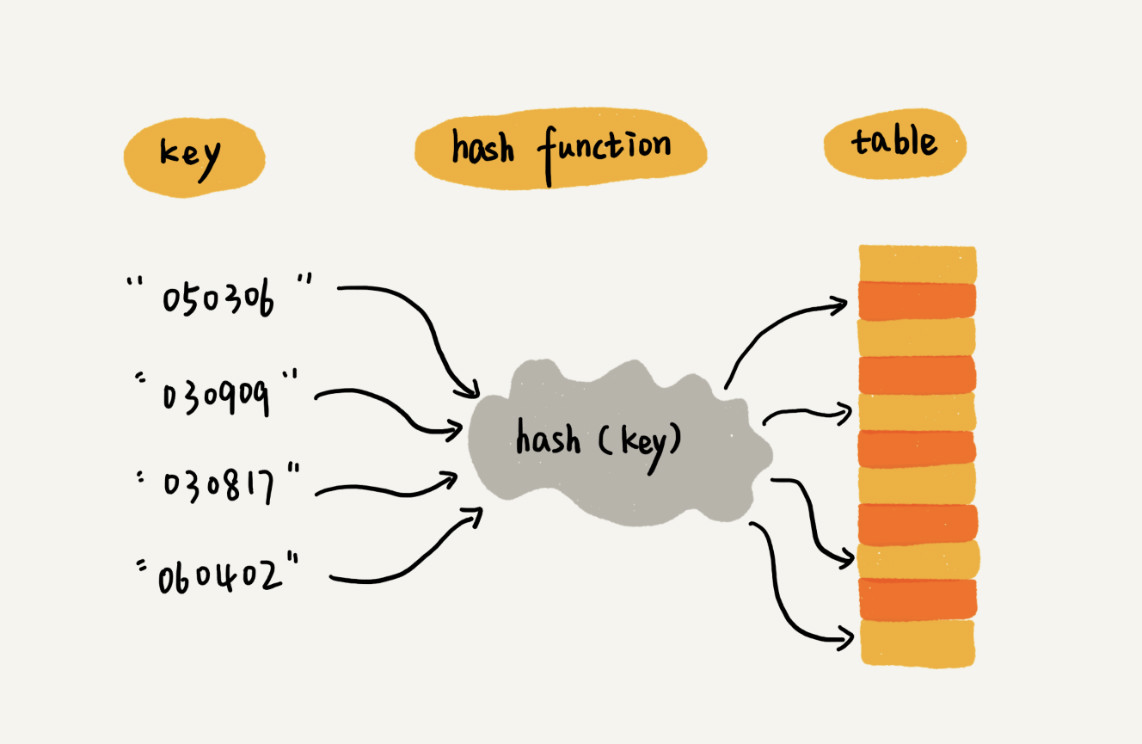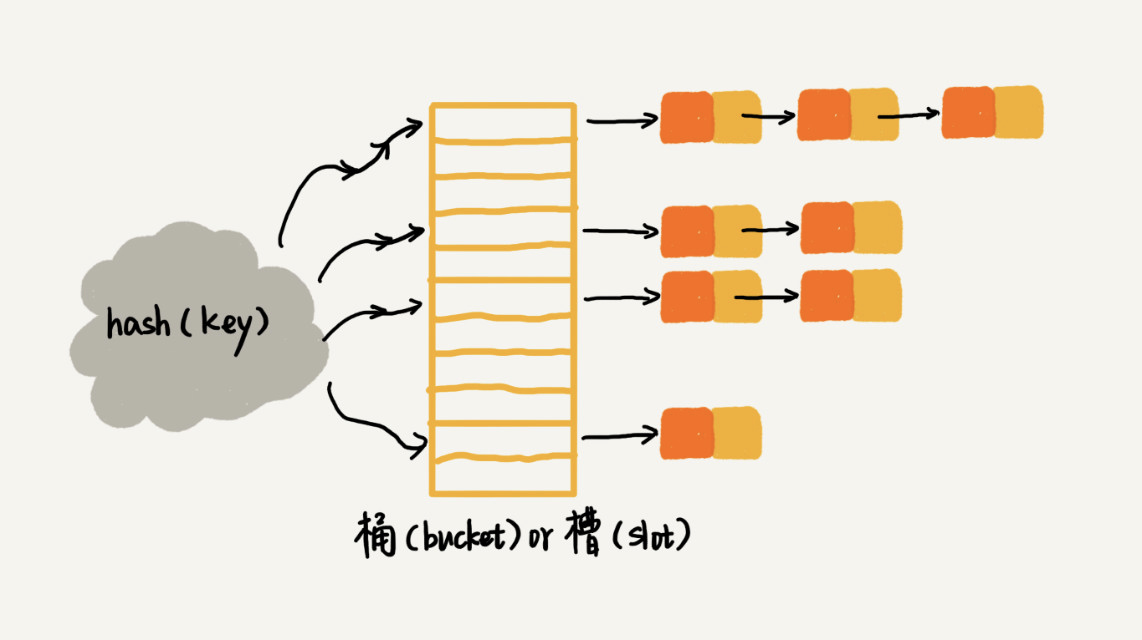# 散列表 -hashTablex-arts# java 中 Object 对象的 hashCode 的实现算法是怎样的

public native int hashCode();

jvm 的实现代码

static inline intptr_t get_next_hash(Thread * Self, oop obj) {  intptr_t value = 0 ;  if (hashCode == 0) {     // This form uses an unguarded global Park-Miller RNG,     // so it's possible for two threads to race and generate the same RNG.     // On MP system we'll have lots of RW access to a global, so the     // mechanism induces lots of coherency traffic.     value = os::random() ;  } else  if (hashCode == 1) {     // This variation has the property of being stable (idempotent)     // between STW operations.  This can be useful in some of the 1-0     // synchronization schemes.     intptr_t addrBits = intptr_t(obj) >> 3 ;     value = addrBits ^ (addrBits >> 5) ^ GVars.stwRandom ;  } else  if (hashCode == 2) {     value = 1 ;            // for sensitivity testing  } else  if (hashCode == 3) {     value = ++GVars.hcSequence ;  } else  if (hashCode == 4) {     value = intptr_t(obj) ;  } else {     // Marsaglia's xor-shift scheme with thread-specific state     // This is probably the best overall implementation -- we'll     // likely make this the default in future releases.     unsigned t = Self->_hashStateX ;     t ^= (t << 11) ;     Self->_hashStateX = Self->_hashStateY ;     Self->_hashStateY = Self->_hashStateZ ;     Self->_hashStateZ = Self->_hashStateW ;     unsigned v = Self->_hashStateW ;     v = (v ^ (v >> 19)) ^ (t ^ (t >> 8)) ;     Self->_hashStateW = v ;     value = v ;  }

0 == Lehmer random number generator,

1 == "somehow" based on memory address

2 ==  always 1

3 ==  increment counter

4 == memory based again("somehow")

1. 随机数

2. 基于内存地址生成

3. 固定值：1，用来测试

4. 自增

5. 利用位移生成随机数

5 的话使用的是 XOR-Shift algorithm  算法生成。  这个算法是伪随机数生成的算法。具体算法介绍可以查看

# HashTable（散列表）## 装载因子## 评论